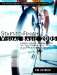# Basic Operators

Visual Basic includes several basic operators that let you do what your code really wants to do: manipulate data. To use them, just dial zero from your phone. No, wait, those operators let you place operator-assisted calls for only \$2.73 for the first minute. The Visual Basic operators let you perform mathematical, logical, bitwise, and string management functions, all at no additional cost.

The most basic operator is the assignment operator, represented by the equals sign (=). You've already seen this operator in use in this chapter. Use it to assign some value to a variable; whatever appears to the right of the operator gets assigned to the reference type or value type variable on the left. The statement:

`fiveSquared = 25 `

assigns a value of 25 to the variable fiveSquared.

Most operators are binary operatorsthey operate on two distinct values, one to the operator's left and one to the right; the result is a single calculated value. It's as if the calculation is fully replaced by the calculated result. For instance, the addition operation:

`seven = 3 + 4 `

becomes:

`seven = 7 `

before the final application of the assignment (=) operator. A unary operator appears just to the left of its operand. For instance, the unary negation operator turns a positive number into a negative number.

`negativeSeven = -7 `

I'll comment on each operator in detail in Chapter 6. But we'll need a quick summary for now so we can manipulate data before we get to that chapter. Table 2-2 lists the main Visual Basic operators and briefly describes the purpose of each one.

##### Table 2-2. Visual Basic Operators

Operator

Description

+

+

The unary plus operator retains the sign of a numeric value. It's not very useful until you get into operator overloading, something covered in Chapter 12, "Operator Overloading."

-

The subtraction operator subtracts the second operand from the first.

-

The unary negation operator reverses the sign of its associated numeric operand.

*

The multiplication operator multiplies two numeric values together.

/

The division operator divides the first numeric operand by the second, returning the quotient including any decimal remainder.

\

The integer division operator divides the first numeric operand by the second, returning the quotient, but with the decimal remainder truncated.

Mod

The modulo operator divides the first numeric operand by the second, and returns only the remainder as an integer value.

^

The exponentiation operator raises the first operand (the base) to the power of the second (the exponent).

&

The string concatenation operator joins two string operands together, and returns a new string with the combined results.

And

The conjunction operator returns True if both Boolean operands are also True.

AndAlso

This operator is just like the And operator, but it doesn't examine or process the second operand if the first one is False.

Or

The disjunction operator returns True if either of the operands is also True.

OrElse

This operator is just like the Or operator, but it doesn't examine or process the second operand if the first one is True.

Not

The negation operator returns the opposite of a Boolean operand.

Xor

The exclusive or operator returns True if exactly one of the operands is also True.

<<

The shift left operator shifts the individual bits in an integer operand to the left by the number of bit positions in the second operand.

>>

The shift right operator shifts the individual bits of an integer operand to the right by the number of bit positions in the second operand.

=

The equal-to comparison operator returns True if the operands are "equal" to each other.

<

The less-than comparison operator returns True if the first operand is "less than" the second.

<=

The less-than-or-equal comparison operator returns True if the first operand is "less than or equal to" the second.

>

The greater-than comparison operator returns True if the first operand is "greater than" the second.

>=

The greater-than-or-equal comparison operator returns True if the first operand is "greater than or equal to" the second.

<>

The not-equal-to comparison operator returns True if the first operand is "not equal to" the second.

Like

The pattern comparison operator returns True if the first operand matches a string pattern specified by the second operand.

Is

The object equal-to comparison operator returns True if both operands truly represent the same instance of a data value in memory. Setting the second operand to Nothing lets you test a reference variable to see if it contains data or not.

IsNot

The object not-equal-to comparison operator is the opposite of the Is operator.

The And, Or, Not, and Xor operators also work as "bitwise" operators. I'll talk about that in the "Operators" section of Chapter 6.

As powerful as operators are, even more power comes when you combine them. This works because any of the operands can be a complex expression that includes its own operands. Parentheses grouped around clauses in operands ensure that values are processed in the order you expect.

`circleArea = pi * (radius ^ 2) `

In this statement, the second operand of the * multiplication operator is another expression, which includes its own operator.Start-to-Finish Visual Basic 2005: Learn Visual Basic 2005 as You Design and Develop a Complete Application
ISBN: 0321398009
EAN: 2147483647
Year: 2006
Pages: 247
Authors: Tim Patrick